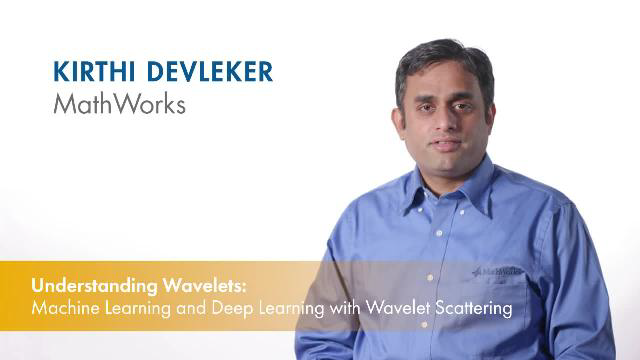# Get Started with Wavelet Toolbox

Perform time-frequency and wavelet analysis of signals and images

Wavelet Toolbox™ provides apps and functions for the time-frequency analysis of signals and multiscale analysis of images. You can denoise and compress data, and detect anomalies, change-points, and transients. The toolbox enables data-centric artificial intelligence (AI) workflows by providing time-frequency transforms and automated feature extractions, including scattering transforms, continuous wavelet transforms (scalograms), Wigner-Ville distribution, and empirical mode decomposition. You can extract edges and oriented features from images using wavelet, wavelet packet, and shearlet transforms.

The apps let you interactively perform time-frequency analysis, signal denoising, or image analysis, and generate MATLAB® scripts to reproduce or automate your work.

You can generate C/C++ and CUDA® code from toolbox functions for embedded deployment.

## VideosUnderstanding Wavelets, Part 1: What Are Wavelets
Explore the fundamental concepts of wavelet transforms in this introductory MATLAB Tech Talk. This video covers what wavelets are and how you can use them to explore your data in MATLAB. The video focuses on two important wavelet transform concepts: scaling and shifting. The concepts can be applied to 2-D data such as images.Understanding Wavelets, Part 2: Types of Wavelet Transforms
Explore the workings of wavelet transforms in detail. You will learn more about the continuous wavelet transforms and the discrete wavelet transform. You will also learn important applications of using wavelet transforms with MATLAB.Understand Wavelets, Part 3: An Example Application of the Discrete Wavelet Transform
Learn how to use to wavelets to denoise a signal while preserving its sharp features in this MATLAB Tech Talk. This video outlines the steps involved in denoising a signal with the discrete wavelet transform using MATLAB. Learn how this denoising technique compares with other denoising techniques.Understanding Wavelets, Part 4: An Example Application of the Continuous Wavelet Transform
Explore a practical application of using continuous wavelet transforms in this MATLAB Tech Talk. Get an overview of how to use MATLAB to obtain a sharper time-frequency analysis of a signal with the continuous wavelet transform. This video uses an example seismic signal to highlight the frequency localization capabilities of the continuous wavelet transform.Understanding Wavelets, Part 5: Machine Learning and Deep Learning with Wavelet Scattering
Wavelet scattering networks help you automatically obtain low-variance features from signals and images for use in machine learning and deep learning applications. In this MATLAB Tech Talk, learn about the wavelet scattering transform and how it can be used as an automatic robust feature extractor for classification.